## Tables index

#### S. Kutluay, N. M. Yagmurlu

International Journal of Partial Differential Equations and Applications. 2017, 5(1), 26-32 doi:10.12691/ijpdea-5-1-4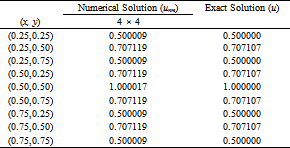• Table 1. Numerical and exact solutions of the model problem for 4 × 4 = 16 elements at t = 0.0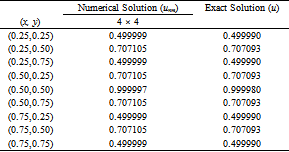• Table 2. Numerical and exact solutions of the model problem for 4 × 4 = 16 elements and Δt = 0.05 at t = 1.0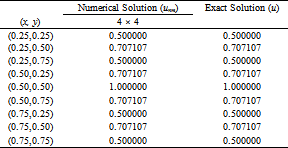• Table 3. Numerical and exact solutions of the problem for 10 × 10 = 100 elements at t = 0.0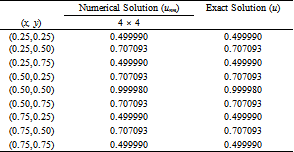• Table 4. Numerical and exact solutions of the problem for 10 × 10 = 100 elements at t = 1.0 with Δt = 0.05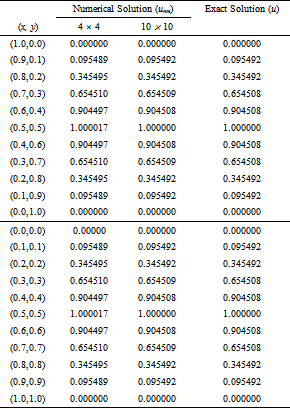• Table 5. Numerical and exact solutions of the problem for 4 × 4 = 16 and 10 × 10 = 100 elements at t = 0.0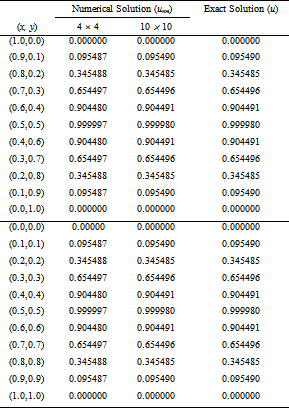• Table 6. Numerical and exact solutions of the problem for 4 × 4 = 16 and 10 × 10 = 100 elements at t = 1.0 with Δt = 0.05• Table 7. Comparison of the error norms L2 and L of the model problem with results from